Data Structures and Algorithms with Object-Oriented Design Patterns in C## Array and Bit-Vector Sets

In this section we consider finite sets over a finite universe. Specifically, the universe we consider is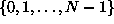, the set of integers in the range from zero to N-1, for some fixed and relatively small value of N.

Let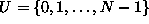be the universe. Every set which we wish to represent is a subset of U. The set of all subsets of U is called the power set  of U and is written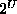. Thus, the sets which we wish to represent are the elements of. The number of elements in the set U, written |U|, is N. Similarly,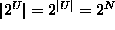. This observation should be obvious: For each element of the universal set U there are only two possibilities: Either it is, or it is not, a member of the given set.

This suggests a relatively straightforward representation of the elements of--an array of bool values, one for each element of the universal set. By using array subscripts in U, we can represent the set implicitly. That is, i is a member of the set if the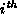array element is true.

Programintroduces the class SetAsArray. The SetAsArray class extends the AbstractSet class defined in Program. This class uses an array of length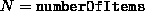to represent the elements ofwhere.Program: SetAsArray fields.Copyright © 2001 by Bruno R. Preiss, P.Eng. All rights reserved.